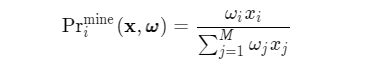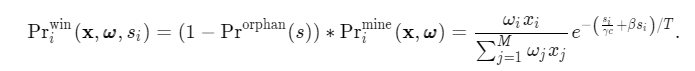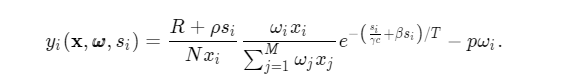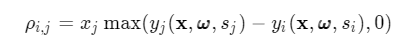区块链网络中矿池选择的演化博弈

Section1-Introduction

1. 一个计算密集型的加密难题解决过程
2. 一个奖励确认过程，用于在矿工发布的区块被网络认可时向矿工提供奖励

Section2-Problem Formulation

涉及参数

• $$N: 区块链网络中独立矿工数量$$
• $$M: 区块链网络中独立矿工形成的矿池数量$$
• $$\mathcal {M}：所有矿池的集合$$
• $$\omega _{i}: 矿池i要求每个独立矿工提供的哈希率$$
• $$\pmb {\omega }: 所有矿池要求每个独立矿工提供的哈希率集合$$
• $$x_{i}: 矿池i中矿工人数占总人数比例$$
• $$\mathbf {x}: 各个矿池中矿工人数占总人数比例的集合$$
• $${\Pr }^{\textrm {mine}}_{i}(\mathbf {x},\pmb {\omega }): 矿池i赢得挖矿竞赛的概率$$
• $$s: 区块大小$$
• $$\gamma: 网络规模相关参数$$
• $$c: 每个链路平均有效信道容量$$
• $$\tau _{p}(s): 大小为s的块的传输延迟时间$$
• $$\beta: 由网络规模和每个节点的平均验证速度共同决定的参数$$
• $$\tau _{v}(s): 验证大小为s的块所需的时间$$
• $${\Pr }^{\textrm {orphan}}(s): 孤立大小为 s 的有效块的概率$$
• $${\Pr }^{\textrm {win}}_{i}(\mathbf {x}, \pmb {\omega }, si): 矿池 i 最终赢得一个大小为si的区块的挖矿竞赛的概率是$$
• $$R: 代币发行的奖励$$
• $$\rho: 每单位数据大小的交易确认价格$$
• $$\rho s_{i}: 区块交易的手续费$$
• $$p: 在 T 期间维持单位哈希率的能源价格$$
• $$p\omega _{i}: 能量成本$$
• $$y_{i}(\mathbf {x}, \pmb {\omega }, s_{i}): 矿工在矿池 i 中的期望收益$$

A.利用POW机制进行挖矿的矿工收益计算

$$\omega _{i}$$

$$\pmb {\omega }=[\omega _{1},\ldots, \omega _{M}]^{\top }$$

$$\mathbf {x}=[x_{1},\ldots,x_{M}]^{\top }$$

$$\mathcal {X}=\left<!–swig￼1–> x_{i}=1}\right}$$$${\Pr }^{\textrm {mine}}_{i}(\mathbf {x},\pmb {\omega })=\frac {\omega {i} x{i}}{\sum _{j=1}^{M}\omega {j} x{j}}$$$$\tau _{p}(s)=\frac {s}{\gamma c}$$

$$\gamma$$

$$\tau _{v}(s)=\beta s$$

$$\beta$$

$$\tau (s)=\tau _{p}(s)+\tau _{v}(s)=\frac {s}{\gamma c}+\beta s.$$

$${\Pr }^{\textrm {orphan}}(s)=1-e^{-\tau (s)/T}=1-e^{-\left({\frac {s}{\gamma c}+\beta s}\right) /T}.$$

$$s_{i}$$

$${\Pr }^{\textrm {win}}{i}(\mathbf {x}, \pmb {\omega }, s{i})=(1-{\Pr }^{\textrm {orphan}}(s))*{\Pr }^{\textrm {mine}}{i}(\mathbf {x},\pmb {\omega })=\frac {\omega {i} x{i}}{\sum {j=1}^{M}\omega {j} x{j}} e^{-\left({\frac {s{i}}{\gamma c}+\beta s{i}}\right)/T}.$$$$\rho$$

$$\rho s_{i}$$

$$p\omega _{i}$$

$$y_{i}(\mathbf {x}, \pmb {\omega }, s_{i})=\frac {R+\rho s_{i}}{N x_{i}}\frac {\omega {i} x{i}}{\sum {j=1}^{M}\omega {j} x{j}} e^{-\left({\frac {s{i}}{\gamma c}+\beta s_{i}}\right)/T}-p\omega _{i}.\qquad$$B.矿池选择过程中的演化博弈

$$\mathcal {G}=\langle \mathcal {N}, \mathcal {M}, \mathbf {x}, {y_{i}(\mathbf {x}, \pmb {\omega }, s_{i})}_{i\in \mathcal {M}} \rangle$$

• $$其中\mathcal {N}表示个体矿工人数,并且\vert \mathcal {N}\vert =N$$
• $$\mathcal {M}={1, 2, \ldots, M}表示所有矿池的集合$$
• $$\mathbf {x}\in \mathcal {X}表示人口状态向量$$
• $${y_{i}(\mathbf {x}, \pmb {\omega }, s_{i})}_{i\in \mathcal {M}}表示每个矿池中单个矿工收益的集合$$

$$\rho {i,j}=x{j}\max (y_{j}(\mathbf {x}, \pmb {\omega }, s_{j}) - y_{i}(\mathbf {x}, \pmb {\omega }, s_{i}), 0)$$补充知识

抗ASIC

ASIC是专用继承电路的缩写，是为特定目的而生产的微芯片，在加密货币领域，ASIC最常用于计算密码难题，特别是用于挖矿过程。